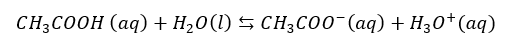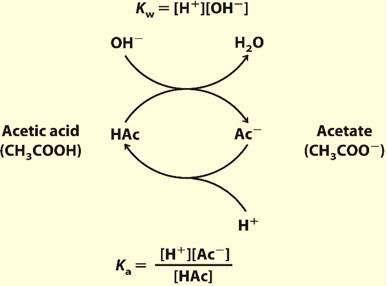# Henderson Hasselbach Equation

Ionic Equilibrium: Henderson-Hasselbach Equation

The shape that weak acid takes in the titration curve when reacted with a weak base can be predicted using the Henderson-Hasselbach equation. The Henderson Hasselbalch equation comes from solving a generic acid problem where both an acid and its conjugate base are present.

You can use this equation to solve problems whenever the 5% approximation is valid This equation simply restates the expression for the ionization of a weak acid, HA, as shown in the following derivation:

Ka = [H+] [A] / [HA]

Solving for [H+], the equation relates to equilibrium constant;[H+] = Ka [HA] /[A].

Then take the negative logarithm of both sides

-log [H+] = -log Ka – log [HA] / [A]

After substituting pKa into the equation for pH, it becomes :pH = pKa – log [HA] / [A]

Finally, the Henderson-Hasselbach equation results after mathematical manipulation of the log term on the right, namelyExamination of this equation shows that

[A]/[HA] = 10/1 when pH = pKa + 1

[A]/[HA] = 1/1 when pH = pKa (titration curve midpoint)

[A]/[HA] = 1/10 when pH = pKa– 1

When [Base] = [Acid], pH = pKa

Recall that A is the conjugate base of HA, while HA is its conjugate acid and the tho quantities make the conjugate acid-base pair.

The Henderson-Hasselbalch equation can be used to calculate pKa, given pH and the molar ratio of proton acceptor and donor in solution. The equation can be arithmetically manipulated to calculate any unknown quantity given logarithmic rules are followed.

The Henderson Hasslebalch is mainly used for buffer system. A Buffer is made of a weak acid and its weak conjugate base present in similar amounts.It is an aqueous solution that tends to resist changes in pH when small amounts of a strong acid (H+) or strong base (OH) are added in the solution. An optimum buffer contains equal amounts of the conjugate acid and conjugate base of the weak acid in the solution. As shown from titration curves, pH changes are the smallest at the midpoint of the titration curve (plateau region) where [HA] = [A] on the addition of acid or base. At this point, the pH = pKafor the weak acid.

Since the buffer systems have both HA and A species, they can absorb either a strong base or strong acid as illustrated for acetic acid in figure 2. When an acid or base is added to the buffer there occurs a small change in the ratio of the conjugate base and conjugate acid. This causes a small movement of the pH.However, the sum of the buffer components in the solution does not change even though their ratio changes.Most buffers are only useful within 1 pH unit of pKa.Fig : Graph range for a general buffer

Consider the following example:Added OH reacts with CH3COOH and the equilibriumshifts to the right. Added H3O+ reacts with CH3COO and the equilibrium shifts to the left.Fig : Ionization of a Buffer solution

.